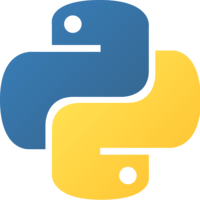1×
Become a member
to unlock all featuresLevel Up!

Access all courses & lessons on egghead today and lock-in your price for life.

Autoplay

Create a Fully Connected TensorFlow Neural Network with Keraspython
^3.0.0

We will set up Keras using Tensorflow for the back end, and build your first neural network using the Keras Sequential model api, with three Dense (fully connected) layers. This network will take in 4 numbers as an input, and output a single continuous (linear) output.

Getting StartedCodeYou must be a Member to view code

Access all courses and lessons, track your progress, gain confidence and expertise.

Become a Member
and unlock code for this lessonDiscussTranscript

Instructor: Start by checking that you have Python installed. Then install TensorFlow and the Keras API. Configure Keras to use TensorFlow as the back-end, then we can make a file to define our neural net. Let's call this one neuralnet.pi.

In neuralnet.pi, first import Sequential from keras.models. Sequential is the Keras name for a neural net that has a linear stack of layers between the input and output, which just means there's no loops and no extra input or output nodes.

Also, import Dense from keras.layers. A Dense Keras layer is a standard, fully-connected layer. We'll be stacking multiple Dense layers together to make our network. A Dense neural network in Keras is called a model.

We'll start by making a new Sequential model. To determine the proper structure of our layers, we first need to know about the shape of our inputs and outputs. For this example, we'll be feeding in a series of four floating point numbers, and we'll be asking the network to predict or calculate the mean of those numbers. We have four numbers as inputs and we expect a single number as the output.

Let's start by making our first Dense layer. The first parameter to Dense is the number of hidden nodes in that layer. There are no hard-and-fast rules to creating network layers, so you'll have to try out different things and see what works for your data.

In general though, a larger number of hidden nodes will create a more complex network that will solve a greater number of problems. However, the more hidden nodes you add, the longer the network will take to train. Large networks may also be prone to over-fitting.

We'll start with a number that is larger than the number of inputs but still small enough to be manageable, which will be eight for this first layer. We will also want to provide a non-linear activation for this layer.

There are many activations you can choose from, like Tanh, or sigmoid. We will choose the reLU activation because it can reduce network training time, and it has been shown to be effective in a large number of practical applications.

Finally, for this first Dense layer only, we have to specify the input dimensions. Keras can't automatically detect what our input is before it compiles the model.

We will tell it that we're going to provide four numbers as our input by setting the input-dim parameter. Now we have our first layer defined, so we can add it to the model.

We will just use the add method, which will stack the new layer onto the model. We can copy that line to add our next layer to the network. We don't need to provide the input dimensions here since it's not an input layer. Then we have to pick the number of nodes in this layer.

Again, there is no hard-and-fast rules. It's common to have a network which grows in size towards the middle and then shrinks back down towards the output.

We'll increase the number of nodes here to 16 and we'll keep the reLU activation. We can copy that to add another layer. This will be our last hidden layer before the output. I'll shrink the hidden nodes back down to eight, and we'll have another relu activation.

Finally, we can copy that last layer to define our output layer. We got to pick the number of nodes in the hidden layers to whatever we wanted. The output layer nodes are defined by the size of the output, which we want to be a single number. That means we only need to define a single node in this layer.

We don't want the non-linear, relu activation here. Instead we just want a single continuous number as the output. We can tell Keras that by specifying the linear activation, which just means we'll get the raw output here.

Now we've defined our entire network, including three hidden layers that will take the four numbers as input and will provide a single number as the output. The last step is to tell Keras that we're done, by telling it to compile the model. We do that by calling the compile method.

There are two parameters that we want to specify, which are the Optimizer and Loss. Just like the activation function, there are many Optimiziers to choose from, like SGD, or RMSprop. We'll choose Adam for this network.

Adam is an Optimizer that performs quite well on a variety of real-world use cases. It may be important to try other Optimizers to see what best fits your data.

Again, for the Loss function there are many to choose from. We're trying to get as close as we can to a particular number as our output, we'll use the common means squared error Loss function. Now in only six lines of code, we've defined our entire network.

The ability to define complex networks in such a small amount of code is one of the most powerful features of the Keras API.